# Identify the Odd One Out using Direction, Position and Number

In this worksheet, students will identify an image that does not fit in with the rest of the group. The focus will be on direction, position and number, so students will need to look at where shapes are, which direction they are facing, and how many there are.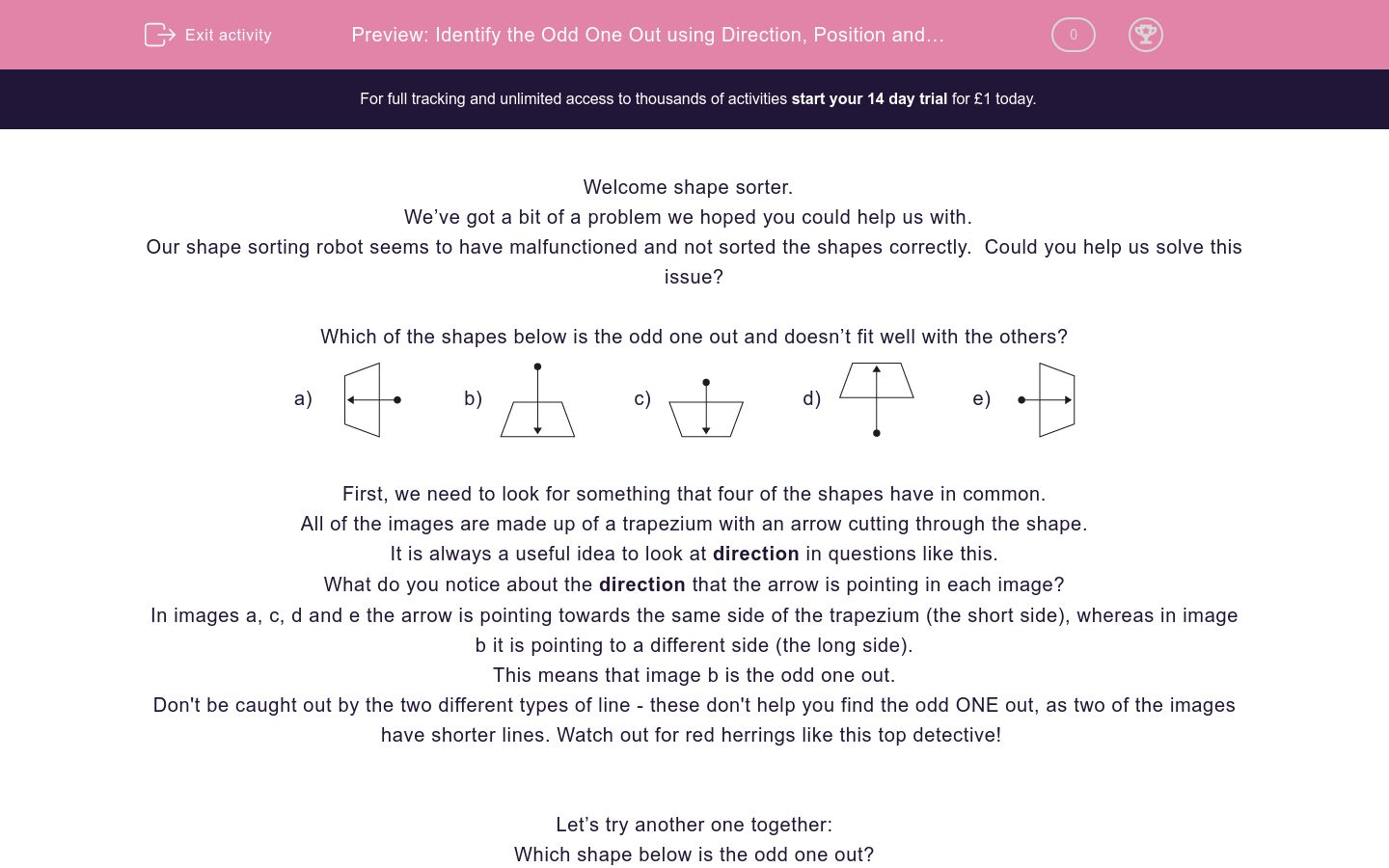Key stage:  KS 2

Curriculum topic:  Spatial and Non-Verbal Reasoning

Curriculum subtopic:  Odd One Out

Difficulty level:### QUESTION 1 of 10

Welcome shape sorter.

We’ve got a bit of a problem we hoped you could help us with.

Our shape sorting robot seems to have malfunctioned and not sorted the shapes correctly.  Could you help us solve this issue?

Which of the shapes below is the odd one out and doesn’t fit well with the others?

a)b)c)d)e)First, we need to look for something that four of the shapes have in common.

All of the images are made up of a trapezium with an arrow cutting through the shape.

It is always a useful idea to look at direction in questions like this.

What do you notice about the direction that the arrow is pointing in each image?

In images a, c, d and e the arrow is pointing towards the same side of the trapezium (the short side), whereas in image b it is pointing to a different side (the long side).

This means that image b is the odd one out.

Don't be caught out by the two different types of line - these don't help you find the odd ONE out, as two of the images have shorter lines. Watch out for red herrings like this top detective!

Let’s try another one together:

Which shape below is the odd one out?

a)b)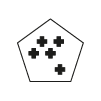c)d)e)First, we need to look for something that four of the shapes have in common.

In questions like this, counting is really important. What could we count?

We have two things to count here: the number of mini shapes and the number of sides of the main shape.

Image a is made up of a 3-sided shape with 3 crosses inside.

Image b is a 5-sided shape with 5 crosses inside.

Image c is a 4-sided shape with 4 crosses inside.

Image d is a 6-sided shape with 5 crosses inside.

Image e is a 4-sided shape with 4 crosses inside.

This means that image d is the odd one out as the number of sides of the main shape does not match the number of mini shapes inside, unlike the other options.

It’s now your turn to identify the odd image.

Remember to look at the direction and position of shapes within the image and always see if there is something you can count.

Good luck shape sorter!

Which of the images below is the odd one out?

a)b)c)d)e)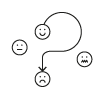Which of the images below is the odd one out?

a)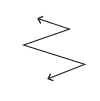b)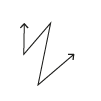c)d)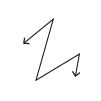e)Which of the images below is the odd one out?

a)b)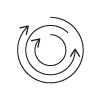c)d)e)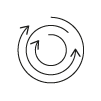Which of the images below is the odd one out?

a)b)c)d)e)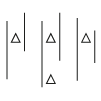Which of the images below is the odd one out?

a)b)c)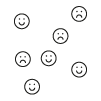d)e)Which of the images below is the odd one out?

a)b)c)d)e)Which of the images below is the odd one out?

a)b)c)d)e)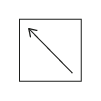Which of the images below is the odd one out?

a)b)c)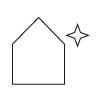d)e)Which of the images below is the odd one out?

a)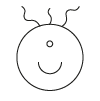b)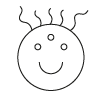c)d)e)Which of the images below is the odd one out?

a)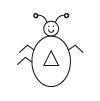b)c)d)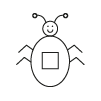e)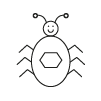• Question 1

Which of the images below is the odd one out?

d)EDDIE SAYS
There are a lot of faces showing different emotions here- I hope you didn't get emotional about it! As is often the case, the arrows here can POINT us to the correct answer. We need to look at where the arrows are coming from and going to. In options a, b, c and e the arrow is going from a happy face to a sad face. In option d the arrow is working in reverse, going from a sad face to a happy face. This makes option d the odd one out.
• Question 2

Which of the images below is the odd one out?

c)EDDIE SAYS
As a detective, there is sometimes more than one route to solve a problem. This is the case in this question, although they both involve counting. We could work out the odd one out by either counting the number of lines making up each arrow or by counting the number of corners in each arrow - both of these options will get us to the correct answer. Options a, b, d and e all have arrows made of 4 lines and 3 corners, whereas option c has an arrow made of 7 lines and 6 corners. This makes option c the odd one out.
• Question 3

Which of the images below is the odd one out?

d)EDDIE SAYS
We have to look carefully at the directions in which the arrows are pointing in this question. In options a, b, c and e there is a clockwise arrow in the middle, followed by an anti-clockwise arrow and then another clockwise arrow on the outside. Phew, there are lots of directions and lines to consider here! In option d there is an anti-clockwise arrow in the middle, then a clockwise, ending with another anti-clockwise. As the pattern is reversed here, this makes option d the odd one out.
• Question 4

Which of the images below is the odd one out?

d)EDDIE SAYS
There is a relationship in action here between the number of triangles and the number of lines. In options a, b, c and e there are two less triangles than the number of lines. For example, option a has 5 lines and 3 triangles and option b has 3 lines and 1 triangle. This is not the case in option d, which has an equal number of lines and triangles (2), making it the odd one out.
• Question 5

Which of the images below is the odd one out?

d)EDDIE SAYS
Here are some more faces showing a lot of emotions! There is a connection between the number of happy faces and the number of sad faces. In options a, b, c and e there is one less sad face than happy face. In option d there are an equal amount of sad and happy faces which does not fit with the rule. This makes option d the odd one out.
• Question 6

Which of the images below is the odd one out?

d)EDDIE SAYS
There are lots of things you could consider here, but you need to find the one that is the top clue and will enable you to reach the correct answer. There is a relationship between the number of sides of the main shape and the numbers of T's inside each. Option a has 7 sides and 4 T's inside. Option b has 5 sides and 2 T's inside. These both have 3 less T's than they do sides. You can test this relationship with the other options, and it works for c and e. It does not work for option d, which has 6 sides and 4 T's inside. This isn't three less T's than sides but 2, so this makes option d the odd one out.
• Question 7

Which of the images below is the odd one out?

a)EDDIE SAYS
It's time to switch into direction detective mode for this question! Each option has a square with an arrow inside. Where are the arrows pointing to? In options b, c, d and e the arrows are pointing to a corner of the square, but in option a they are pointing to a side. This makes option a the odd one out. Don't get SQUARE eyes detective - just 3 questions to go!
• Question 8

Which of the images below is the odd one out?

c)EDDIE SAYS
Here you need to spot the relationship between the number of sides of the big shapes and the number of points on the star outside each. Can you work it out? Option a has a 3-sided shape with a 3-pointed star on the outside. Option b has a 4-sided shape with a 4-pointed star outside. The number of sides of the main shape appears to match the number of points on the star. Can you test this with the other options? If you do, you will find that options d and e also follow this pattern but option c does not. Option c has a 5-sided shape with a 4-pointed star which doesn't match and makes it the odd one out.
• Question 9

Which of the images below is the odd one out?

d)EDDIE SAYS
There are a few bad hair days going on here for these aliens! Can you spot the connection between the number of strands of hair and the number of eyes? In options a, b, c and e there are 2 more strands of hair than the number of eyes. For example, the alien in option a has 1 eye and 3 strands of hair. This connection is not maintained in option d, which has 3 strands of hair and 2 eyes, which makes it the odd alien out!
• Question 10

Which of the images below is the odd one out?

c)